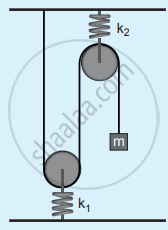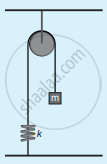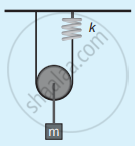Tamil Nadu Board of Secondary EducationHSC Science Class 11th

# Tamil Nadu Board Samacheer Kalvi solutions for Class 11th Physics Volume 1 and 2 Answers Guide chapter 10 - Oscillations [Latest edition]

#### Chapters## Chapter 10: Oscillations

Evaluation
Evaluation [Pages 218 - 219]

### Tamil Nadu Board Samacheer Kalvi solutions for Class 11th Physics Volume 1 and 2 Answers Guide Chapter 10 OscillationsEvaluation [Pages 218 - 219]

#### Multiple Choice Questions

Evaluation | Q I. 1. | Page 218

In a simple harmonic oscillation, the acceleration against displacement for one complete oscillation will be __________.

• an ellipse

• a circle

• a parabola

• a straight line

Evaluation | Q I. 2. | Page 218

A particle executing SHM crosses points A and B with the same velocity. Having taken 3 s in passing from A to B, it returns to B after another 3 s. The time period is ____________.

• 15 s

• 6 s

• 12 s

• 9 s

Evaluation | Q I. 3. | Page 218

The length of a second’s pendulum on the surface of the Earth is 0.9 m. The length of the same pendulum on the surface of planet X such that the acceleration of the planet X is n times greater than the Earth is

• 0.9 n

• 0.9/"n""m"

• 0.9 n2m

• 0.9/"n"^2

Evaluation | Q I. 4. | Page 218

A simple pendulum is suspended from the roof of a school bus which moves in a horizontal direction with an acceleration a, then the time period is

• "T" ∝ 1/("g"^2 + "a"^2)

• "T" ∝ 1/sqrt("g"^2 + "a"^2)

• "T" ∝ sqrt("g"^2 + "a"^2)

• "T" ∝ ("g"^2 + "a"^2)

Evaluation | Q I. 5. | Page 218

Two bodies A and B whose masses are in the ratio 1 : 2 are suspended from two separate massless springs of force constants kA and kB respectively. If the two bodies oscillate vertically such that their maximum velocities are in the ratio 1 : 2, the ratio of the amplitude A to that of B is

• sqrt(("k"_"B")/(2"k"_"A"))

• sqrt(("k"_"B")/(8"k"_"A"))

• sqrt((2"k"_"B")/("k"_"A"))

• sqrt((8"k"_"B")/("k"_"A"))

Evaluation | Q I. 6. | Page 218

A spring is connected to a mass m suspended from it and its time period for vertical oscillation is T. The spring is now cut into two equal halves and the same mass is suspended from one of the halves. The period of vertical oscillation is

• T’ = sqrt2"T"

• T’ = "T"/sqrt2

• T’ = sqrt(2"T")

• T’ = sqrt("T"/2)

Evaluation | Q I. 7. | Page 218

The time period for small vertical oscillations of block of mass m when the masses of the pulleys are negligible and spring constant k1 and k2 is• T = 4π sqrt("m"(1/"k"_1 + 1/"k"_2))

• T = 2π sqrt("m"(1/"k"_1 + 1/"k"_2))

• T = 4π sqrt("m"("k"_1 + "k"_2))

• T = 2π sqrt("m"("k"_1 + "k"_2))

Evaluation | Q I. 8. | Page 219

A simple pendulum has a time period T1. When its point of suspension is moved vertically upwards according to as y = kt2, where y is the vertical distance covered and k = 1 ms−2, its time period becomes T2. Then, T "T"_1^2/"T"_2^2 is (g = 10 ms−2)

• 5/6

• 11/10

• 6/5

• 5/4

Evaluation | Q I. 9. | Page 219

An ideal spring of spring constant k, is suspended from the ceiling of a room and a block of mass M is fastened to its lower end. If the block is released when the spring is un-stretched, then the maximum extension in the spring is

• 4"Mg"/"k"

• "Mg"/"k"

• 2"Mg"/"k"

• "Mg"/(2"k")

Evaluation | Q I. 10. | Page 219

A pendulum is hung in a very high building oscillates to and fro motion freely like a simple harmonic oscillator. If the acceleration of the bob is 16 ms−2 at a distance of 4 m from the mean position, then the time period is

• 2 s

• 1 s

• 2πs

• πs

Evaluation | Q I. 11. | Page 219

A hollow sphere is filled with water. It is hung by a long thread. As the water flows out of a hole at the bottom, the period of oscillation will ____________.

• first increase and then decrease

• first decrease and then increase

• increase continuously

• decrease continuously

Evaluation | Q I. 12. | Page 219

The damping force on an oscillator is directly proportional to the velocity. The units of the constant of proportionality are

• kg ms−1

• kg ms−2

• kg s−1

• kg s

Evaluation | Q I. 13. | Page 219

When a damped harmonic oscillator completes 100 oscillations, its amplitude is reduced to 1/3 of its initial value. What will be its amplitude when it completes 200 oscillations?

• 1/5

• 2/3

• 1/6

• 1/9

Evaluation | Q I. 14. | Page 219

Which of the following differential equations represents a damped harmonic oscillator?

• ("d"^2"y")/"dt"^2 + "y" = 0

• ("d"^2"y")/"dt"^2 + γ"dy"/"dt" + "y" = 0

• ("d"^2"y")/"dt"^2 + "k"^2"y" = 0

• "dy"/"dt" + "y" = 0

Evaluation | Q I. 15. | Page 219

If the inertial mass and gravitational mass of the simple pendulum of length l are not equal, then the time period of the simple pendulum is

• T = 2π sqrt(("m"_"i""l")/("m"_"g""g"))

• T = 2π sqrt(("m"_"g""l")/("m"_"i""g"))

• T = 2π "m"_"g"/"m"_"i" sqrt("l"/"g")

• T = 2π "m"_"i"/"m"_"g" sqrt("l"/"g")

Evaluation [Page 220]

### Tamil Nadu Board Samacheer Kalvi solutions for Class 11th Physics Volume 1 and 2 Answers Guide Chapter 10 OscillationsEvaluation [Page 220]

Evaluation | Q II. 1. | Page 220

What is meant by periodic motion? Give any two examples, for periodic motion.

Evaluation | Q II. 1. | Page 220

What is meant by non-periodic motion? Give any two examples, for non-periodic motion.

Evaluation | Q II. 2. | Page 220

What is meant by the force constant of a spring?

Evaluation | Q II. 3. | Page 220

Define the time period of simple harmonic motion.

Evaluation | Q II. 4. | Page 220

Define the frequency of simple harmonic motion.

Evaluation | Q II. 5. | Page 220

What is an epoch?

Evaluation | Q II. 6. | Page 220

Write short notes on two springs connected in series.

Evaluation | Q II. 7. | Page 220

Write short notes on two springs connected in parallel.

Evaluation | Q II. 8. | Page 220

Write down the time period of simple pendulum.

Evaluation | Q II. 9. | Page 220

State the laws of the simple pendulum?

Evaluation | Q II. 10. | Page 220

Write down the equation of the time period for the linear harmonic oscillator.

Evaluation | Q II. 11. | Page 220

What is meant by free oscillation?

Evaluation | Q II. 12. | Page 220

Explain damped oscillation. Give an example.

Evaluation | Q II. 13. | Page 220

Define forced oscillation. Give an example.

Evaluation | Q II. 14. | Page 220

What is meant by maintained oscillation? Give an example.

Evaluation | Q II. 15. | Page 220

Explain resonance. Give an example.

Evaluation [Page 220]

### Tamil Nadu Board Samacheer Kalvi solutions for Class 11th Physics Volume 1 and 2 Answers Guide Chapter 10 OscillationsEvaluation [Page 220]

Evaluation | Q III. 1. | Page 220

What is meant by simple harmonic oscillation? Give examples and explain why every simple harmonic motion is a periodic motion whereas the converse need not be true.

Evaluation | Q III. 2. | Page 220

Describe Simple Harmonic Motion as a projection of uniform circular motion.

Evaluation | Q III. 3. | Page 220

What is meant by angular harmonic oscillation? Compute the time period of angular harmonic oscillation.

Evaluation | Q III. 4. | Page 220

Write down the difference between simple harmonic motion and angular simple harmonic motion.

Evaluation | Q III. 5. | Page 220

Discuss the simple pendulum in detail.

Evaluation | Q III. 6. | Page 220

Explain the horizontal oscillations of a spring.

Evaluation | Q III. 7. | Page 220

Describe the vertical oscillations of a spring.

Evaluation | Q III. 8. | Page 220

Write short notes on the oscillations of the liquid column in the U-tube.

Evaluation | Q III. 9. | Page 220

Discuss in detail the energy in simple harmonic motion.

Evaluation | Q III. 10. | Page 220

Explain in detail the four different types of oscillations.

Evaluation [Pages 220 - 221]

### Tamil Nadu Board Samacheer Kalvi solutions for Class 11th Physics Volume 1 and 2 Answers Guide Chapter 10 OscillationsEvaluation [Pages 220 - 221]

#### Numerical Problems

Evaluation | Q IV. 1. | Page 220

Consider the Earth as a homogeneous sphere of radius R and a straight hole is bored in it through its centre. Show that a particle dropped into the hole will execute a simple harmonic motion such that its time period is

T = 2π sqrt("R"/"g")

Evaluation | Q IV. 2. | Page 220

Consider a simple pendulum of length l = 0.9 m which is properly placed on a trolley rolling down on a inclined plane which is at θ = 45° with the horizontal. Assuming that the inclined plane is frictionless, calculate the time period of oscillation of the simple pendulum.

Evaluation | Q IV. 3. | Page 220

A piece of wood of mass m is floating erect in a liquid whose density is ρ. If it is slightly pressed down and released, then executes simple harmonic motion. Show that its time period of oscillation is T = 2π sqrt("m"/("Ag"ρ))

Evaluation | Q IV. 4. | Page 221

Consider two simple harmonic motion along the x and y-axis having the same frequencies but different amplitudes as x = A sin (ωt + φ) (along x-axis) and y = B sin ωt (along y-axis). Then show that

"x"^2/"A"^2 + "y"^2/"B"^2 - (2"xy")/"AB" cos φ = sin^2 φ

and also discuss the special cases when

1. φ = 0
2. φ = π
3. φ = π/2
4. φ = π/2 and A = B
5. φ = π/4

Note: when a particle is subjected to two simple harmonic motions at right angle to each other the particle may move along different paths. Such paths are called Lissajous figures.

Evaluation | Q IV. 5. | Page 221

Show that for a particle executing simple harmonic motion.

1. the average value of kinetic energy is equal to the average value of potential energy.
2. average potential energy = average kinetic energy = 1/2 (total energy)

Hint: average kinetic energy = <kinetic energy> = 1/"T" int_0^"T" ("Kinetic energy") "dt" and

average potential energy = <potential energy> = 1/"T" int_0^"T" ("Potential energy") "dt"

Evaluation | Q IV. 6. | Page 221

Compute the time period for the following system if the block of mass m is slightly displaced vertically down from its equilibrium position and then released. Assume that the pulley is light and smooth, strings and springs are light.## Chapter 10: Oscillations

Evaluation## Tamil Nadu Board Samacheer Kalvi solutions for Class 11th Physics Volume 1 and 2 Answers Guide chapter 10 - Oscillations

Tamil Nadu Board Samacheer Kalvi solutions for Class 11th Physics Volume 1 and 2 Answers Guide chapter 10 (Oscillations) include all questions with solution and detail explanation. This will clear students doubts about any question and improve application skills while preparing for board exams. The detailed, step-by-step solutions will help you understand the concepts better and clear your confusions, if any. Shaalaa.com has the Tamil Nadu Board of Secondary Education Class 11th Physics Volume 1 and 2 Answers Guide solutions in a manner that help students grasp basic concepts better and faster.

Further, we at Shaalaa.com provide such solutions so that students can prepare for written exams. Tamil Nadu Board Samacheer Kalvi textbook solutions can be a core help for self-study and acts as a perfect self-help guidance for students.

Concepts covered in Class 11th Physics Volume 1 and 2 Answers Guide chapter 10 Oscillations are Oscillations, Simple Harmonic Motion (SHM), Angular Simple Harmonic Motion, Linear Simple Harmonic Oscillator, Energy in Simple Harmonic Motion, Types of Oscillations.

Using Tamil Nadu Board Samacheer Kalvi Class 11th solutions Oscillations exercise by students are an easy way to prepare for the exams, as they involve solutions arranged chapter-wise also page wise. The questions involved in Tamil Nadu Board Samacheer Kalvi Solutions are important questions that can be asked in the final exam. Maximum students of Tamil Nadu Board of Secondary Education Class 11th prefer Tamil Nadu Board Samacheer Kalvi Textbook Solutions to score more in exam.

Get the free view of chapter 10 Oscillations Class 11th extra questions for Class 11th Physics Volume 1 and 2 Answers Guide and can use Shaalaa.com to keep it handy for your exam preparation